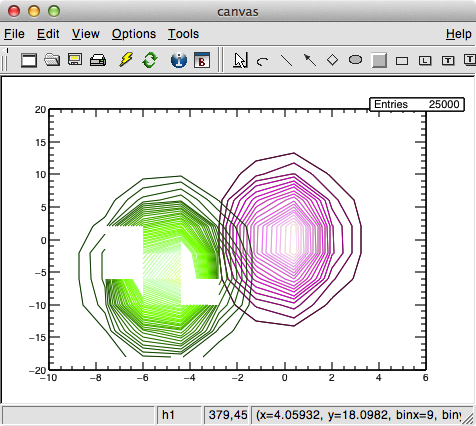# Put color to a contour?

Hi,

Anybody have a idea of how I can put a define color (for example green or the color of my preference) for a contour plot, I mean put color to h->Draw(“CONT1”), instead of the automatic color

Thanks

Nicolás

Hi.

Sounds like you want to change the colormap. The default colourmap in ROOT is the rainbow colour

``gStyle->SetPalette(1)``

I guess you can change to other palette by changing the above argument (from 1 to which ever number available). Now to define your own colormap, have a look at this website
rcnp.osaka-u.ac.jp/~noji/colormap

OR

root.cern.ch/drupal/content/how … or-palette

rcnp.osaka-u.ac.jp/~noji/colormap

Thats a nice collection of palette s ! could you provide also the value you pass to TColor::CreateGradientColorTable for each of them ?

Note also the new palette 57 in ROOT: root.cern.ch/drupal/content/rai … or-map#r36

I mean, If I have:

``````See->Draw("CONT1");
Semu->Draw("CONT1 SAME");
``````

How I can set different colors for each contour?

Ah, should have been much clearer with your question, and save time in getting answersHave a look at code below for a “long and dirty way” to get what you want.

``````void plot_magenta();
void plot_green();

int test(){

TCanvas *c  = new TCanvas("c","canvas",0,0,800,600);

// Create two 2D histograms for testing
TH2F *h1 = new TH2F("h1","",10,-10,6,10,-20,20);
TH2F *h2 = new TH2F("h2","",10,-10,6,10,-20,20);
Float_t px, py;
for (Int_t i = 0; i < 25000; i++) {
gRandom->Rannor(px,py);
h2->Fill(px,5*py);
h1->Fill(px-5,5*py-5);

}

// must have these lines - I don't know why
TExec *ex1 = new TExec("ex1","plot_magenta();");
TExec *ex2 = new TExec("ex2","plot_green();");

// must draw it once here
h2->Draw("cont1");

// call first color then draw first contour plot again
ex1->Draw();
h2->Draw("cont1 same");

// call second colormap then draw second contour plot on top of previous
ex2->Draw();
h1->Draw("cont1 same");

return 1;
}

// define colormap for first contour plot
void plot_magenta() {
const UInt_t Number = 4;
Double_t Red[Number]    = {0.30, 1.00, 1.00, 1.00};
Double_t Green[Number]  = {0.00, 0.00, 0.40, 0.90};
Double_t Blue[Number]   = {0.10, 1.00, 1.00, 1.00};
Double_t Length[Number] = {0.00, 0.30, 0.60, 1.00};
const Int_t nb=50;
gStyle->SetNumberContours(nb);
return;
}

// define second colormap for the other contour plot
void plot_green() {
const UInt_t Number = 4;
Double_t Red[Number]    = {0.00, 0.40, 0.80, 0.90};
Double_t Green[Number]  = {0.20, 1.00, 1.00, 1.00};
Double_t Blue[Number]   = {0.00, 0.00, 0.80, 0.60};
Double_t Length[Number] = {0.00, 0.40, 0.80, 1.00};
const Int_t nb=50;
gStyle->SetNumberContours(nb);
return;
}
``````Ok, thanks a lot!!

Dear useROOT,

We like a lot the palette you display here: rcnp.osaka-u.ac.jp/~noji/colormap
It would be great to introduce them ROOT.
For each of them, Would it be possible to have the value you passed to TColor::CreateGradientColorTable ?